# Bounds on gaps between primes

It is a long-standing unsolved problem to prove that there is always a prime between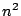and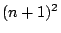. This is equivalent to showing that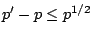. Since the average size of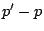is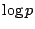, and it is conjectured that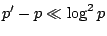, current results seem to be very far from the final truth.

Goldston and Heath-Brown [ MR 85e:11064] have shown that the pair correlation conjecture implies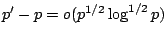.

Problem: Find a believable conjecture about the zeros of the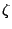-function which implies that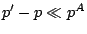for all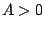. Even the case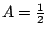would be significant.

For an example of a non-believable conjecture which may imply that there are small gaps between consecutive primes, see the article on the Alternative Hypothesis.

Heath-Brown [ MR 83m:10078] showed that if Montgomery's conjecture on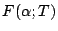holds in some neighborhood of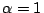then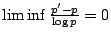. The proof only requires the continuity ofat. This continuity also follows from the alternative hypothesis, so there may be hope of proving this unconditionally.

Erdös used sieve methods to show that there exists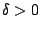such that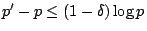for a positive proportion of primes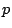. (It would be helpful if someone could provide details on the history of this problem and an up-to-date account of current results).

It has not yet been shown that there is a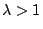such that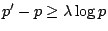for a positive proportion of.

Back to the main index for L-functions and Random Matrix Theory.